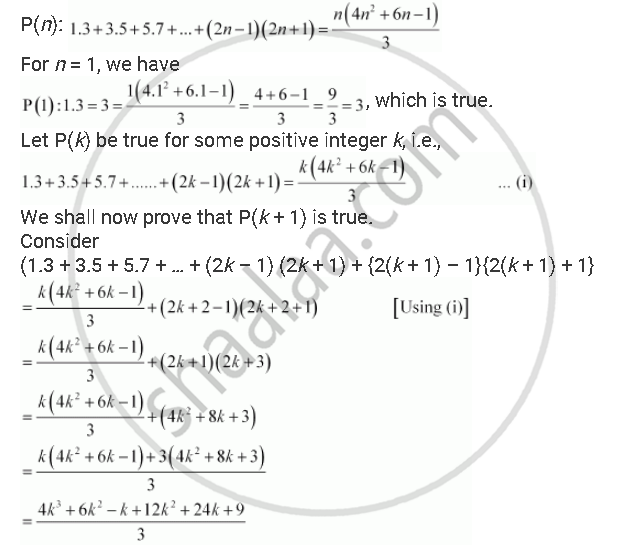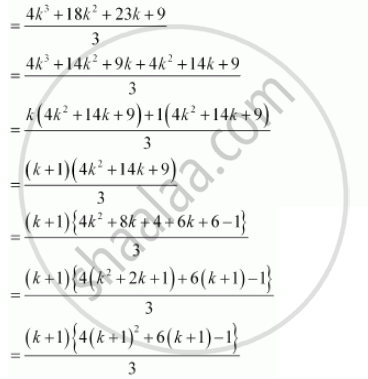# Prove the Following by Using the Principle of Mathematical Induction for All N ∈ N: 1.3 + 3.5 + 5.7 + ...+(2n -1)(2n + 1) = (N(4n^2 + 6n -1))/3 - Mathematics

Prove the following by using the principle of mathematical induction for all n ∈ N

1.3 + 3.5 + 5.7 + ...+(2n -1)(2n + 1) = (n(4n^2 + 6n -1))/3

#### Solution

Let the given statement be P(n), i.e.,Thus, P(k + 1) is true whenever P(k) is true.

Hence, by the principle of mathematical induction, statement P(n) is true for all natural numbers i.e., n.

Concept: Principle of Mathematical Induction
Is there an error in this question or solution?

#### APPEARS IN

NCERT Class 11 Mathematics
Chapter 4 Principle of Mathematical Induction
Q 7 | Page 94